×#### Thank you for registering.

One of our academic counsellors will contact you within 1 working day.

Click to Chat

1800-1023-196

+91-120-4616500

CART 0

• 0

MY CART (5)

Use Coupon: CART20 and get 20% off on all online Study Material

ITEM
DETAILS
MRP
DISCOUNT
FINAL PRICE
Total Price: Rs.

There are no items in this cart.
Continue Shopping• Complete JEE Main/Advanced Course and Test Series
• OFFERED PRICE: Rs. 15,900
• View Details

Chapter 1: Rational Numbers Exercise – 1.3

Question: 1

Subtract the first rational number from the second in each of the following: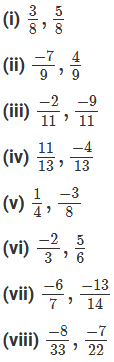Solution: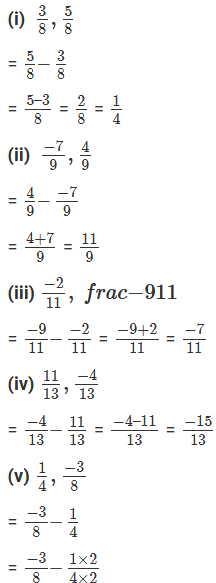Question: 2

Evaluate each of the following:Solution: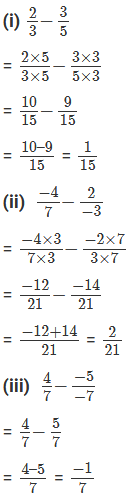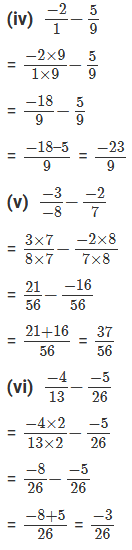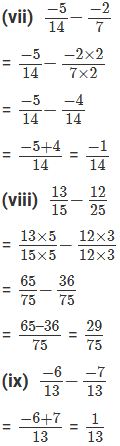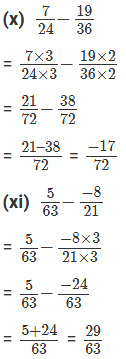Question: 3

The sum of the two numbers is 5/9. If one of the numbers is 1/3, Find the others.

Solution:

It is given that:

The sum of the two numbers is 5/9.

One of the number is 13

Let, the other number be x.Question: 4

The sum of the two numbers is -1/3. If one of the number is −12/3, Find the others.

Solution:

It is given that: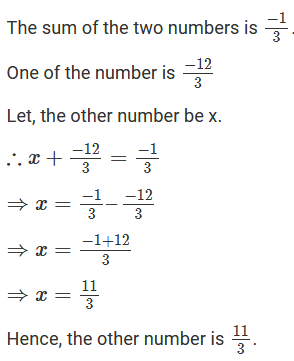Question: 5

The sum of the two numbers is −4/3. If one of the number is -5, Find the others.

Solution:

It is given that:Question: 6

The sum of the two rational numbers is -8. If one of the number is −15/7, Find the others.

Solution:

It is given that:

The sum of the two numbers is – 8.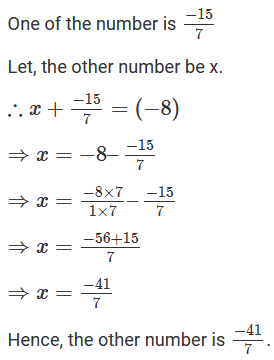Question: 7

What should be added to −7/8 so as to get 5/9?

Solution:

It is given that: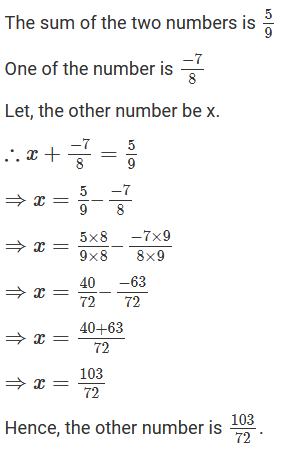Question: 8

What number should be added to −5/11 so as to get 26/33?

Solution:

It is given that: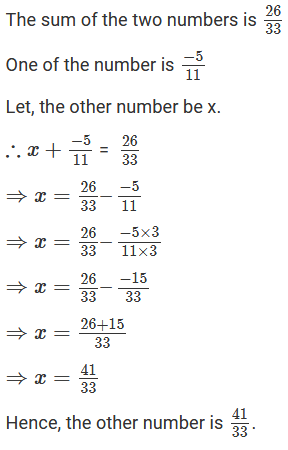Question: 9

What number should be added to −5/7 to get −2/3?

Solution:

It is given that: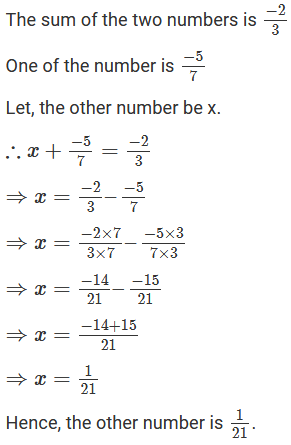Question: 10

What number should be subtracted from −5/3 to get 5/6?

Solution:

It is given that: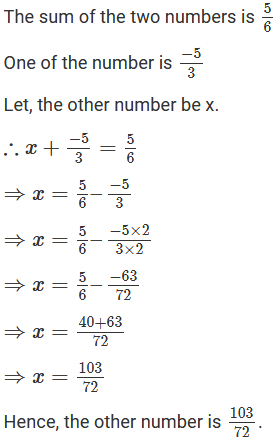Question: 11

What number must be subtracted from 3/7 to get 5/4?

Solution:

It is given that: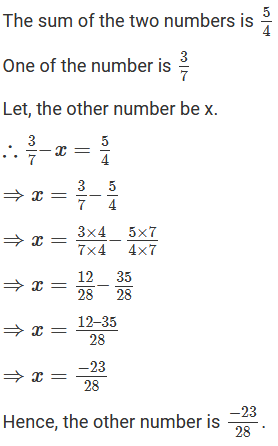Question: 12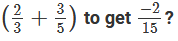Solution:

It is given that:Question: 13

What should be added to (1/2 + 1/3 + 1/5) to get 3?

Solution:

It is given that: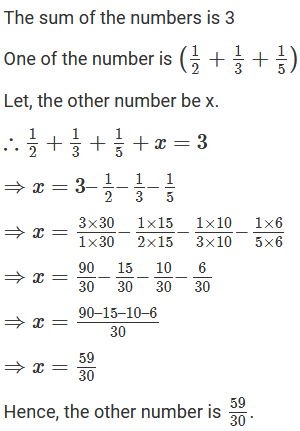Question: 14

What should be subtracted from (3/4 -2/3) to get −1/6?

Solution:

It is given that:Question: 15

Fill in the blanks:Solution: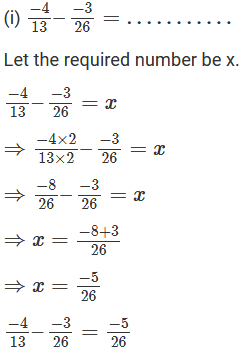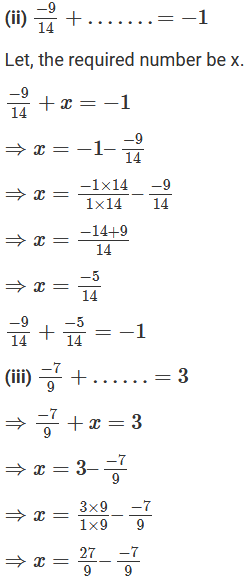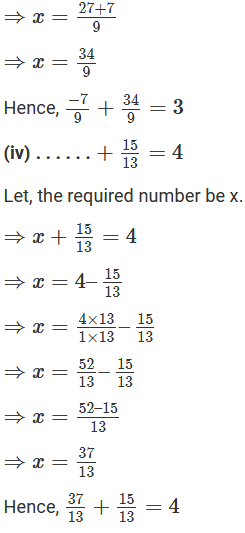### Course Features

• 728 Video Lectures
• Revision Notes
• Previous Year Papers
• Mind Map
• Study Planner
• NCERT Solutions
• Discussion Forum
• Test paper with Video Solution